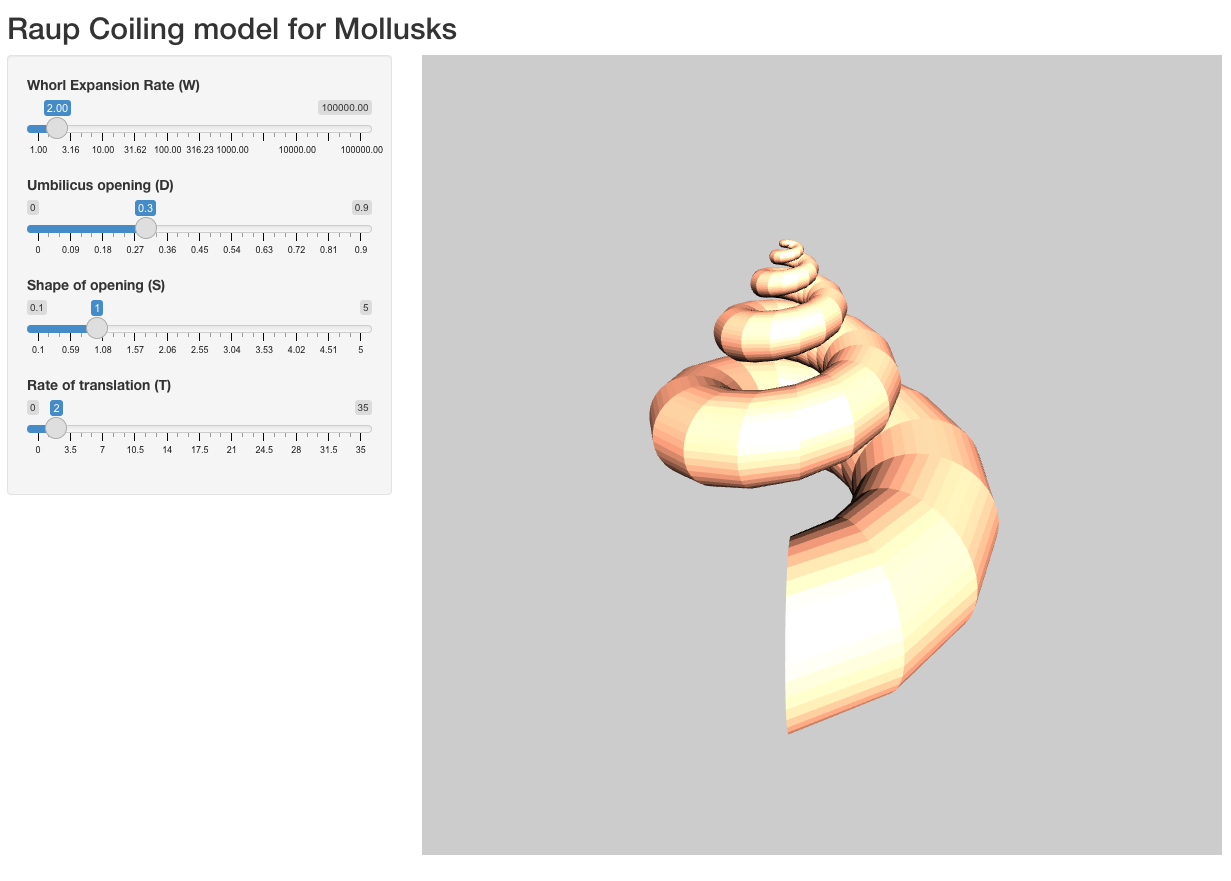raupShiny

2021-09-01

As a follow-up on the previous post, I finally got the time to write a simplified version of the shiny app we made for the exhibition on biomineralization at the Museum für Naturkunde in Magdeburg, so that people can built upon it and make a better, more complex version, and so I am going to briefly explain it here.
``````library(shiny)
library(shinyWidgets)
library(rgl)

make_elliptic_generating_shape <- function(D,S,res=100){
#Let's define the original ray as 1
a <- 1
rc <- (D+1)*a/(1-D)
t<-seq(0,2*pi,by=pi/res)
b <- a/S
circle_0 <- cbind(r=rc + a*cos(t), y= b*sin(t), phi=0)
return(circle_0)
}

#Function to coil the shape around the axis
coiling <- function(RT,W,generating_shape, turns,steps,dir="dextral"){
PHI <- seq(0,2*pi*turns,length=steps)
far_end <- generating_shape[1,1]
closest_end <- approx(generating_shape[generating_shape[,1]<far_end-1,2],
generating_shape[generating_shape[,1]<far_end-1,1],0)\$y
D <- closest_end/far_end
rc <- (D+1)/(1-D)
rho <- function(theta, W, r0) r0 * W^(theta/(2*pi))
y <- function(y0,W,theta,rc,T) y0 * W^(theta/(2*pi)) + rc*T*(W^(theta/(2*pi))-1)
circle <- apply(generating_shape,1,
function(x)lapply(PHI,
function(theta)cbind(r=rho(theta, W, x['r']),
y=y(x['y'],W,theta, rc,RT),
phi=theta)))
circle <- do.call(rbind,lapply(circle,function(x)do.call(rbind,x)))

#To cartesian coordinates
if(dir=="dextral"){
XYZ <- list(X = circle[,1] * sin(circle[,3]),
Y = circle[,1] * cos(circle[,3]),
Z = circle[,2])
}else{
XYZ <- list(X = circle[,1] * cos(circle[,3]),
Y = circle[,1] * sin(circle[,3]),
Z = circle[,2])
}
XYZ
}

#Function to create the indices of the vertices of the mesh
pt_n<- rep(1:(res*2+1),each=steps)
step <- 1:steps
eg <- expand.grid(1:(2*res),1:(steps-1))
apply(eg,1,function(x)c(which(step==x & pt_n==x),
which(step==x+1 & pt_n==x),
which(step==x+1 & pt_n==x+1),
which(step==x & pt_n==x+1)))
}``````

OK that first part is the one I showed last time, with an additional function `pt2quad` which takes a dataframe of points (as XYZ coordinates) and return a dataframe of quadrilaterals that will define the 3D shape.

``````JS.logify <-"function logifySlider (sliderId) {
// regular number style
\$('#'+sliderId).data('ionRangeSlider').update({
'prettify': function (num) { return (Math.pow(10, num).toFixed(2)); }
})
}"
setTimeout(function() {
logifySlider('W')
}, 5)})"

uirgl <- fluidPage(
titlePanel("Raup Coiling model for Mollusks"),
sidebarLayout(
sidebarPanel(
sliderInput(input="W",label="Whorl Expansion Rate (W)",
min = 0, max = 5, value = 0.301, step = .0001),
sliderInput(input="D",label="Umbilicus opening (D)",
min=0, max=0.9,value=0.3,step=0.01),
sliderInput(input="S",label="Shape of opening (S)",
min=0.1, max=5,value=1,step=0.01),
sliderInput(input="RT",label="Rate of translation (T)",
min=0, max=35,value=2,step=0.1),
),
mainPanel(
rglwidgetOutput("coilrgl",width=800, height=800)
)
)
)``````

`shiny` makes it very easy! This is the whole code to create the actual HTML page the user is going to interact with. We just define our variables while creating sliders for variable selection. I already discussed the two javascript functions before.

``````serverrgl <- function(input,output){
mqd <- reactive({ #Compute the shape
# First we need to grab the variables picked by the user
RT <- input\$RT
D <- input\$D
res <- 20 #Resolution of a single step is fixed here
W <- round(10^input\$W,2)
S <- input\$S
# To make the app more functional we're actually computing the number of turns instead of
# proposing it as a variable.
turns <- 5
if(W<1.5 & RT>2) turns <- 10
if(D>0.5) turns <- 10
if(D<0.1 & RT==0) turns <- 2
if(W>100) turns <- 1
# We also made the number of steps a function of the number of turns:
steps <- ifelse(turns>5,150,75)
# Then we compute the generating shape
circle <- make_elliptic_generating_shape(D,S,res)
# We coil it:
ce <- coiling(RT,W,circle,turns,steps,"dextral")
# I am a bit ashamed of the next 3 lines, but 3D geometry is hard :)
CE <- as.data.frame(ce)
CE <- CE[c(2,3,1)]
CE[,2] <- -1*CE[,2]
# We compute the indices of the vertices
# And we make it a 3D mesh
})
output\$coilrgl <- renderRglwidget({ #Render it as 3D plot
rgl.open(useNULL=TRUE)
# Having the shape being computed outside this function allows the shape not to be recomputed all the time
# (useful in the actual app in which the users modify the angle of the plot as a variable, not so much here):
m <- mqd()
rgl.viewpoint(zoom = 1, theta=90, phi=30)
bg3d(color="grey80")
material3d(color="lightsalmon",emission="black",alpha=1,specular="white",ambient="black",textype="luminance")
shade3d(m,override=TRUE) #Apart from this line that actual plot the shape, the rest is pure aesthetic
light3d(theta=10,phi=10)
rglwidget() #Launch the rgl widget
})
}

#The last line finally launch the app:
shinyApp(ui = uirgl, server = serverrgl,options=list("launch.browser"=TRUE))``````

And this is what happens "server-side". The retrival of the variables, the computation of the shape and its plotting, and that's it. Note the `output\$coilrgl`: "coilrgl" is the name we gave in the previous code block to the placeholder for the rgl plot in the HTML page; and "output" is the name of the server's output... because we named it that way in the arguments of the function (see first line). Both `reactive` and `renderRglwidget` are "reactive" in that they will re-run every time they need to (i. e. when the user do something that would change their output).The result.